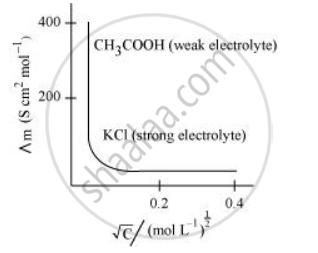Share

# Define Conductivity and Molar Conductivity for the Solution of an Electrolyte. Discuss Their Variation with Concentration. - Chemistry

ConceptConductance of Electrolytic Solutions Measurement of the Conductivity of Ionic Solutions

#### Question

Define conductivity and molar conductivity for the solution of an electrolyte. Discuss their variation with concentration.

#### Solution

Conductivity of a solution is defined as the conductance of a solution of 1 cm in length and area of cross-section 1 sq. cm. The inverse of resistivity is called conductivity or specific conductance. It is represented by the symbolκ. If ρ is resistivity, then we can write:

k = 1/rho

The conductivity of a solution at any given concentration is the conductance (G) of one unit volume of solution kept between two platinum electrodes with the unit area of cross-section and at a distance of unit length.

ie G= k a/l = k.l =k

Since a = 1, l = 1)

Conductivity always decreases with a decrease in concentration, both for weak and strong electrolytes. This is because the number of ions per unit volume that carry the current in a solution decreases with a decrease in concentration.

Molar conductivity:

Molar conductivity of a solution at a given concentration is the conductance of volume V of a solution containing 1 mole of the electrolyte kept between two electrodes with the area of cross-section A and distance of unit length.

Lambda_m = kA/l

Now, l = 1 and A = V (volume containing 1 mole of the electrolyte).

:.Lambda_m = kV

Molar conductivity increases with a decrease in concentration. This is because the total volume V of the solution containing one mole of the electrolyte increases on dilution.

The variation of Lambda_m with sqrtc for strong and weak electrolytes is shown in the following plot:Is there an error in this question or solution?

#### Video TutorialsVIEW ALL 

Solution Define Conductivity and Molar Conductivity for the Solution of an Electrolyte. Discuss Their Variation with Concentration. Concept: Conductance of Electrolytic Solutions - Measurement of the Conductivity of Ionic Solutions.
S